# Partnership - Concept and Problems

Partnership is an important chapter in Quantitative section of IBPS and other banking examination.

When two or more people joins hands with a common goal to attain profits. Every partner invests either time, money or his patents to help partnership firm to reap profits.

There are many problems in partnerships regarding profit shares, investment period etc. I will try to solve one question from each part.

### Question 1

Raj invested Rs 76000 in a business. After few months Monty joined him and invests Rs 57000. At the end of year both of them share the profits at the ratio of 2:1. After how many months Monty joined Raj ?

Solution - We can simply compute per month investment of both partnership

Raj invested Rs 76,000 for 12 months and Monty invested Rs 57,000 for x months.

Now 76000 × 12 / 57000 × x = 2 :1

⇒ 76 × 12 / 2 = 57x

⇒ x = 8

So Monty invested his money for 8 months and he joined after 4 months.

### Question 2

A and B started a business by investing money in ratio of 5:6. C joined them after 6 months by sharing an amount equal to B's share. At the end of year 20% profit was earned which was equal equal to Rs 98,000. How much money was invested by C ?
Solution -
= First of all we will calculate the weighted ratios

⇒ A = 5 × 12 = 60
⇒ B = 6 × 12 = 72
⇒ C = 6 × 6 = 36

Total investment at the end of year = 98000 × 100/20 = Rs 4,90,000

⇒ Investment by C = 490000 × 36 / 168 × 2 = Rs 210000

### Question 3

A, B and C shared profits in ratio of 5:7:8. They partnered for 14 months, 8 months and 7 months respectively. What was he ratio of their investments ?

Solution - Simply multiply profit sharing ratio with investment ratio to get investment amount ratio.

Let X is the total investment

⇒14 x = 5
⇒ 8 x = 7
⇒ 7x = 8

⇒ Final investment ratio = 20 : 49 : 64

### Question 3

Sita and Geeta started a business by investing Rs. 120000 and Rs.135000 respectively. Find the share of each out of an annual profit of Rs. 35700.
Solution :-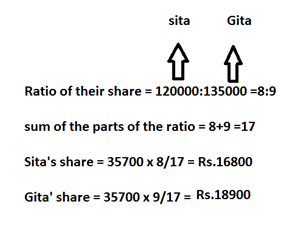• When investors are there for the different duration of  time in the business , then the investments are calculated for  a unit of time by having ( investment x number of units of time) . Now gain or loss is divided in the ratio of these investments.

### Question 4.

Ravi started a business by investing Rs.36000. After 3 months Shayam joined him by investing Rs.36000. Find the share of each in the annual profit of Rs. 37100.
Solution -Now let us do some questions:

### Question 5.

Sony , Mony and Tony start a business each investing Rs.20000. After 5 months Sony withdrew Rs.5000, Mony withdrew Rs.4000 and Tony added Rs.6000 more. At the end of the year, a total profit Of Rs. 69900 was recorded. Find the share of each?
Solution -### Question 6.

Sheela started a business with Rs. 21000 and is joined afterwords by Reema with Rs 36000. After how many months did Reema join if the profits at the end of the year are divided equally?### Question 7.

X and Y invested in a business. They earned some profit which they divided in the ratio of 2:3. If X invested Rs. 40000. Find the amount invested by Y?
Solution -### Question 8.

A , B , C contract a work for Rs .1100.A and B together are to do 7/11 of the work . What is C’s share ?
Solution -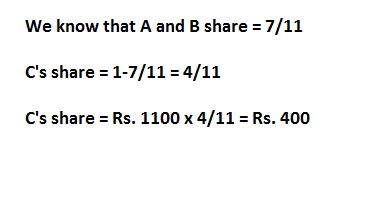### Question 9.

Mohinder and Surinder entered into a partnership investing Rs. 12000 and Rs. 9000 respectively . After 3 months , Sudhir joined them with an investment of Rs.15000. What is the share of Sudhir in a half yearly profit of Rs. 9500?
Solution -### Question 10.

In a partnership , A invests 1/6 of the capital for 1/6 of the time , B invests 1/3 of the capital for 1/3 of the time and C , the rest of the capital for the whole time. What is the share of B in the profit f Rs. 4600.
Solution -### Question 11.

Three partners shared the profit in a business in the ratio 5 : 7 : 8. They had partnered for 14 months, 8 months and 7 months respectively. What was the ratio of their investments?
Solution - Let the first partner invests Rs x for 14 months, second partner invests Rs.y for 8 months, Rs z for 7 months.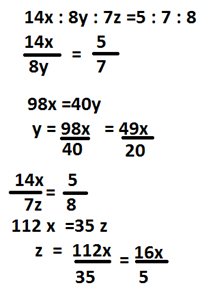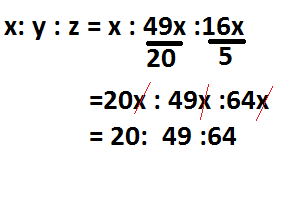### Question 12.

Ajay and Sahil enter into a partnership investing Rs. 50000 and Rs. 40000, respectively. They agree to share profits in the ratio of their capitals. Find the share of Ajay in a profit of Rs. 22500 after one
(a) Rs. 12500
(b) Rs. 9500
(c) Rs 10500
(d) None of these

### Question 13.

Navdeep, Rahul and Raj entered into a partnership Navdeep invested Rs. 16000 for 9 months. Rahul invested Rs. 12000 for 6 months and Raj invested Rs. 8000 for 12 months. At the end of a year there was a profit of Rs. 26000. Find the share of Rahul in the profit.
(a) Rs. 8000
(b) Rs. 7500
(c) Rs. 6000
(d) None of these

### Question 14.

Divya starts business with Rs. 3500 and 5 months after Jyoti joins Divya as her partner. After a year the profits are divided in the ratio of 2:3. How much did Jyoti contribute?
(a) Rs. 7000
(b) Rs. 11000
(c) Rs. 9000
(d) None of these

### Question 15.

Aman began a business with Rs. 550 and was joined afterwards by Rahul with Rs. 330. When did Rahul join if the profits at the end of the year were divided in the ratio 10:3?
(a) After 4 months
(b) After 6 months
(c) After 4.5 months
(d) None of these

### Question 16.

A, B and C invested capitals in the ratio 3:5:9; the timing of their investments being in the ratio 2:3 1. In what ratio would their profits be distributed?
(a) 2:5:3
(b) 3:2:5
(c) 7:5:3
(d) None of these

### Question 17.

A, B and C start a business. If the ratio of their periods of investments are 2:3:6 and their profits are in the ratio of 4:5:6, then the ratio of capitals of A, B and C is
(a) 6:8:10
(b) 12 10:6
(c) 10: 12:6
(d) None of these

### Question 18.

A, B, C and D enter into partnership, A subscribes 1/3 of the capital, B 1/4, C 1/and D the rest. What is the share of D out of a profit of Rs. 6000?
(a) Rs. 2000
(b) Rs. 1600
(c) Rs. 1200
(d) Rs. 1300

### Question 19.

A and B started a business with initial investments in the ratio 5:7. If after one year their profits were in the ratio 1:2 and the period for A's investment was 7 months, B invested the money for
(a) 6 months
(b) 9 months
(c) 10 months
(d) 4 months

### Question 20.

A, B, C enter into a partnership with shares in the 7/24/36/5 ratio After 4 months, A increase his share by 50%. If the total profit at the end of one year be Rs. 21600, then B's share in the profit is
(a) Rs. 2100
(b) Rs. 2400
(c) Rs. 3600
(d) Rs, 4000

### Question 21.

B is a sleeping partner and A working. A puts in Rs.5000 and B puts in 6000, A received 12.5% of profit for managing the business and rest is divided in proportion to their capitals. A's share of profit in a profit of Rs. 880 is
(a) Rs. 350
(b) Rs. 400
(c) Rs. 420
(d) Rs. 460

### Question 22.

A starts business with a capital of Rs. 1200. B and C join with some investments after 3 and 6 months, respectively. If at the end of a year, the profit is divided in the ratio 2:3:5 respectively, what is B's investment in the business?
(a) Rs. 2400
(c) Rs. 3600
(b) Rs. 1800
(d) Rs. 6000

## Solutions

#### Ans. 12. (a)Now Ajay's Share
5/✕ 22500= Rs. 12500

#### Ans. 13. (c)Rahul's Share = 6000.

#### Ans. 14. (c)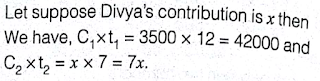Then, Profit for Divya/Profit for Jyoti
5C1 ✕ t1/9C2 ✕ t2

=>2/42000/7x
or, x= 42000✕3/2✕7= Rs. 9000.
Jyoti's Contribution is Rs. 9000.

#### Ans. 15. (b)

Let Rahul remain in the business for x months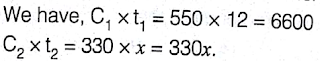Then, Profit for Aman Share of profit/ Rahul Share of profit
= C1 ✕ t1/C2 ✕ t2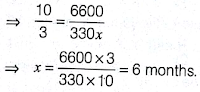#### Ans. 16. (a)#### Ans. 17. (b)

or, 12 : 10 : 16
Thus A, B and C invested their captains in the ratio 12 : 10 : 6.

#### Ans. 22. (a)